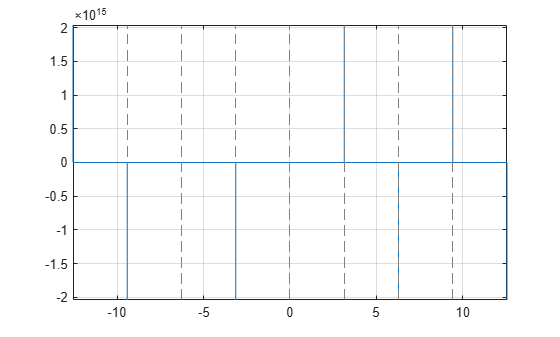# csc

Symbolic cosecant function

## Syntax

``csc(X)``

## Description

example

``csc(X)` returns the cosecant function of `X`.`

## Examples

### Cosecant Function for Numeric and Symbolic Arguments

Depending on its arguments, `csc` returns floating-point or exact symbolic results.

Compute the cosecant function for these numbers. Because these numbers are not symbolic objects, `csc` returns floating-point results.

`A = csc([-2, -pi/2, pi/6, 5*pi/7, 11])`
```A = -1.0998 -1.0000 2.0000 1.2790 -1.0000```

Compute the cosecant function for the numbers converted to symbolic objects. For many symbolic (exact) numbers, `csc` returns unresolved symbolic calls.

`symA = csc(sym([-2, -pi/2, pi/6, 5*pi/7, 11]))`
```symA = [ -1/sin(2), -1, 2, 1/sin((2*pi)/7), 1/sin(11)]```

Use `vpa` to approximate symbolic results with floating-point numbers:

`vpa(symA)`
```ans = [ -1.0997501702946164667566973970263,... -1.0,... 2.0,... 1.2790480076899326057478506072714,... -1.0000097935452091313874644503551]```

### Plot Cosecant Function

Plot the cosecant function on the interval from $-4\pi$ to $4\pi$.

```syms x fplot(csc(x),[-4*pi 4*pi]) grid on```### Handle Expressions Containing Cosecant Function

Many functions, such as `diff`, `int`, `taylor`, and `rewrite`, can handle expressions containing `csc`.

Find the first and second derivatives of the cosecant function:

```syms x diff(csc(x), x) diff(csc(x), x, x)```
```ans = -cos(x)/sin(x)^2 ans = 1/sin(x) + (2*cos(x)^2)/sin(x)^3```

Find the indefinite integral of the cosecant function:

`int(csc(x), x)`
```ans = log(tan(x/2))```

Find the Taylor series expansion of `csc(x)` around ```x = pi/2```:

`taylor(csc(x), x, pi/2)`
```ans = (x - pi/2)^2/2 + (5*(x - pi/2)^4)/24 + 1```

Rewrite the cosecant function in terms of the exponential function:

`rewrite(csc(x), 'exp')`
```ans = 1/((exp(-x*1i)*1i)/2 - (exp(x*1i)*1i)/2)```

### Evaluate Units with `csc` Function

`csc` numerically evaluates these units automatically: `radian`, `degree`, `arcmin`, `arcsec`, and `revolution`.

Show this behavior by finding the cosecant of `x` degrees and `2` radians.

```u = symunit; syms x f = [x*u.degree 2*u.radian]; cosecf = csc(f)```
```cosecf = [ 1/sin((pi*x)/180), 1/sin(2)]```

You can calculate `cosecf` by substituting for `x` using `subs` and then using `double` or `vpa`.

## Input Arguments

collapse all

Input, specified as a symbolic number, variable, expression, or function, or as a vector or matrix of symbolic numbers, variables, expressions, or functions.

collapse all

### Cosecant Function

The cosecant of an angle, α, defined with reference to a right angled triangle isThe cosecant of a complex argument, α, is

`$\text{csc}\left(\alpha \right)=\frac{2i}{{e}^{i\alpha }-{e}^{-i\alpha }}\text{\hspace{0.17em}}.$`